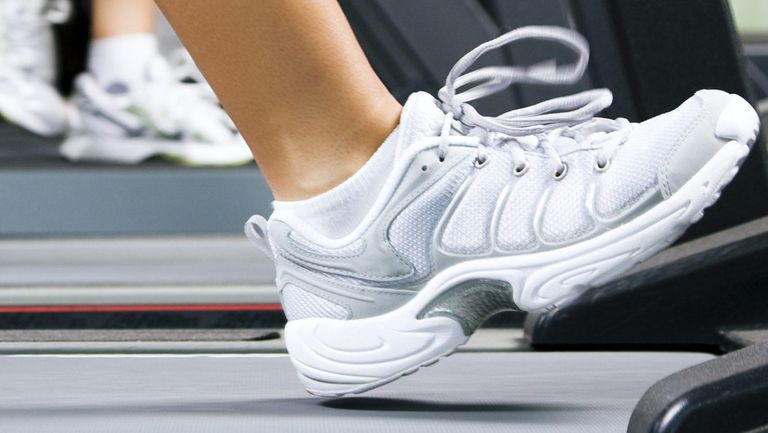# How to calculate the number of calories burned walking

Time to get the calculator out!Researchers may have discovered that spending an hour in a hot bath can burn as many calories as a 30-minute walk. But, tempting as it may be, spending half your life in the tub probably isn’t practical.

But just how many calories do you burn walking? Many of us now track every step we take, but what does that step count really mean? Ready to find out exactly how many calories are burned walking? Before you lace up your best women's walking shoes, you just need our handy formula to help take out the guesswork.

Calorie-counting apps are all well and good, but few give you more than a passing indication of the number of calories used walking. Why? Because some of the most commonly used formulas fail to account for individual factors that affect the rate at which we burn calories. This includes details such as height and, in some cases, weight.

“As a very general thought, you can burn between 100-300 calories in 30 minutes from walking,” says Vikash Sharma, a Doctor of Physical Therapy at New York City’s Perfect Stride Physical Therapy. “Climbing hills, walking at a faster pace or using intervals in your walking routine? Then you are sure to increase the number of calories you burn.”

Here's everything you need to know to calculate the number of calories burned walking.

## Why you may be burning more calories than you think

The good news? You could be burning more calories than you think, especially if you’re on the petite side. Researchers who analysed multiple data sets published over the past 50 years discovered that standard calculations underestimated calorie burn in 97% of cases. Plus, your height makes more of a difference than it’s been given credit for.

“The taller you are, the less energy it takes to walk a mile,” says Peter Weyand, the study’s co-author, who helped to come up with the new formula. “This means the rate at which you burn calories while walking at the same speed is slower compared with someone who is shorter.”

The formula measures the number of calories burned per minute during level walking and works for all ages. To use it, all you’ll need to know your walking speed. Haven’t got one of the best fitness trackers? Try the freeMap My Walkapp. This can record the speed and duration of your walks, plotting them on a nifty interactive map.

## How to calculate the number of calories burned walking

Here’s the formula you need to know:

Calories burned per minute = (0.035 * body weight in kg) + ((Velocity in m/s ^ 2) / Height in m)) * (0.029) * (body weight in kg)

Don't worry – it’s not as complicated as it looks. Let’s say you’re 1.6m tall, weigh 60kg and typically walk at 1.4 metres per second (that's about 3.1mph).

1. Multiply your body weight in kilograms (i.e. 60) by 0.035. Eg. 0.035 x 60 = 2.1
2. Square your velocity (or speed) in metres per second, i.e. multiply it by itself. Eg. 1.4 x 1.4 = 1.96
3. Divide the result by your height in metres (i.e. 1.6). Eg.1.96 ÷ 1.6 = 1.225

The formula now looks like this:

Calories burned per minute = (2.1) + ((1.96)/1.6)) * (0.029) * (60)

or

Calories burned per minute = (2.1) + (1.225) * (0.029) * (60)

2.1 + 1.225 = 3.325

3.325 x 0.029 = 0.096425

0.096425 x 60 = 5.7855

This means that someone of your height and weight will typically burn 5.8 calories per minute when walking on a level surface at a speed of 1.4m/s or 3.1mph. A standard formula accounting for weight alone would give you an estimated figure of 4 calories burned per minute.

While the formula can’t account for gradient or fitness levels, scientists claim it is much more accurate than the algorithms typically used by calorie-burn calculators, treadmills and fitness apps.